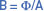# Magnetic Flux Density (B)

Want create site? Find Free WordPress Themes and plugins.

Magnetic Flux density is the measure of the number of magnetic lines of force per unit of cross-sectional area.

The general symbol for magnetic flux density is B and the unit is the weber per square meter (Wb/m2). One weber per square meter is called a tesla (T).

If both the total flux and the area of the magnetic path are known, the flux density is found from:Where:

• B = flux density in tesla (Wb/m2)
• Φ = total flux in webers
• A = area in m2

## Magnetic Flux Density Example

A magnetic circuit has a cross-sectional area of 100mm2 and a flux density of 0.01T. Calculate the total flux in the circuit.Note: The answer is expressed in webers and not in lines of force.

## Example of Magnetic Flux Density 2

An air core coil has 0.65 μ Wb of flux in its core. Calculate the flux density if the core diameter is 4 cm.

Solution

First, we’ll calculate the core area:

$A=\pi ~{{r}^{2}}=3.14*{{\left( 0.02m \right)}^{2}}=1.256*~{{10}^{-3}}~{{m}^{2}}$

Now, we can calculate the magnetic flux density using the following formula:

$B=\frac{\varphi }{A}=~\frac{0.65*~{{10}^{-6}}~Wb}{1.256*~{{10}^{-3}}~{{m}^{2}}}=5.175*~{{10}^{-4}}~T$

Did you find apk for android? You can find new Free Android Games and apps.